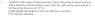# Elastic Collision: Solving Components of the Problem

• harisf
We know kinetic energy is conserved:2(2km/s)2 + 1(0m/s)2 = 2(v1'cos10)2 + v2'28 - 2(v1'cos10)2 = v2'2 (our second equation) Then sub in equations into each other for v2'.f

#### harisf

1. Homework Statement
The problem is in the picture(attached)

2. Homework Equations
I know we have to use
m1v1 + m2v2 = m1v1' + m2v2'
(1/2)m1v1^2 + (1/2)m2v2^2 = (1/2)m1v1'^2 + (1/2)m2v2'^2

3. The Attempt at a Solution
All I know is that it needs to be split up into components.

#### Attachments

•ps.jpg
19 KB · Views: 339
Welcome to PF!

Welcome to PF!(please type the question in future … it's much easier to read than having to look at a picture in a separate window)

(and try using the X2 and X2 tags just above the Reply box)

I know we have to use
m1v1 + m2v2 = m1v1' + m2v2'
(1/2)m1v1^2 + (1/2)m2v2^2 = (1/2)m1v1'^2 + (1/2)m2v2'^2

All I know is that it needs to be split up into components.

Yes, the first equation is a vector equations, and needs to be split up into two scalar equations.

(You could choose West and North for the component directions, for example.)

So … show us what you get.Health Warning:This problem can get very messy unless you translate it into
the reference frame in which the c of m is stationary.

David

Health Warning:This problem can get very messy unless you translate it into
the reference frame in which the c of m is stationary.

David

No, you should be able to do it in the reference frame given.

Thanks guys, and yes I will try this question out. I got some help today from a friend, he said that there was no need for NS/EW components. He told me to make a triangle. So therefore the resulting velocity after the collision of the 2amu mass would be v1'cos10.

2(2km/s) + 1(0m/s) = 2(v1'cos10) + v2'
4 - 2v1'cos10 = v2' (this will be equation one)

We know kinetic energy is conserved:
2(2km/s)2 + 1(0m/s)2 = 2(v1'cos10)2 + v2'2
8 - 2(v1'cos10)2 = v2'2 (our second equation)

Then sub in equations into each other for v2'.
I have to go for now but I will get back to you guys soon on the problem. Please keep in mind that I am only in grade 12. Thanks again.